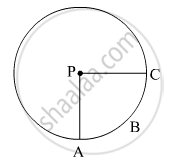# In the Given Figure, If A(P-abc) = 154 Cm2 Radius of the Circle is 14 Cm, Find (1) ∠ a P C (2) L ( Arc Abc) . - Mathematics

#### Question

In the given figure, if A(P-ABC) = 154 cm2  radius of the circle is 14 cm, find

(1) ∠APC

(2) l ( arc ABC) .#### Solution

The radius of the circle, r = 14 cm
(1)
A(P-ABC) = Area of the sector PABC = 154 cm2

$\therefore \frac{\theta}{360° } \times \pi r^2 = 154$

$\Rightarrow \frac{\angle APC}{360° } \times \frac{22}{7} \times \left( 14 \right)^2 = 154$

$\Rightarrow \angle APC = \frac{154 \times 7 \times 360° }{22 \times 196} = 90°$

Thus, the measure of ∠APC is 90º.

(2) l (arc ABC) = Length of the arc ABC

$= \frac{\angle APC}{360°} \times 2\pi r = \frac{90° }{360° } \times 2 \times \frac{22}{7} \times 14$  = 22 cm

Thus, the length of arc ABC is 22 cm.

Is there an error in this question or solution?

#### Video TutorialsVIEW ALL 

In the Given Figure, If A(P-abc) = 154 Cm2 Radius of the Circle is 14 Cm, Find (1) ∠ a P C (2) L ( Arc Abc) . Concept: Perimeter and Area of a Circle.